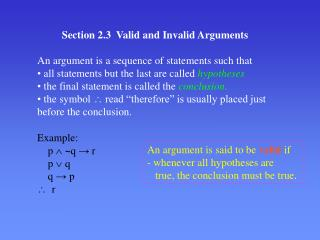Download PresentationSection 2.3 Valid and Invalid Arguments

# Section 2.3 Valid and Invalid Arguments - PowerPoint PPT PresentationDownload Presentation## Section 2.3 Valid and Invalid Arguments

- - - - - - - - - - - - - - - - - - - - - - - - - - - E N D - - - - - - - - - - - - - - - - - - - - - - - - - - -
##### Presentation Transcript

1. Section 2.3 Valid and Invalid Arguments • An argument is a sequence of statements such that • all statements but the last are called hypotheses • the final statement is called the conclusion. • the symbol  read “therefore” is usually placed just before the conclusion. Example: p ~q → r p  q q → p  r An argument is said to bevalid if - whenever all hypotheses are true, the conclusion must be true.

2. Example of a valid argument (form) p  (q  r) ~ q  p  r

3. An invalid argument p → q ~r q → p  r  p → r Invalid row

4. Tautology - is a statement (form) that is always true regardless of the truth values of the individual statement variables. • Examples: • p ~p (eg. the number n is either > 0 or  0 ) • p  q → p • (p → q → r) → (p → r) We need to study tautologies because any valid argument is equivalent to a tautology. In particular, every theorem we have proved is a tautology.

5. Example: p  (q  r) ~q  p  r is a valid argument, [p  (q  r)]  [~ q] → [p  r] is a tautology. In other words, an argument H1 H2 … Hn  Conclusion is valid if and only if H1 H2 … Hn→ conclusion is a tautology.

6. Two most important valid argument forms Modus Ponens : means method of affirming p → q p  q Example: If n 5, then n! is divisible by 10. n= 7 7! is divisible by 10. Modus Tollens : means method of denying p → q ~q ~p Example: If n is odd, then n2 is odd. n2 is even. n is even.

7. More valid forms Conjunctive simplification: p  q  p Example: The function f is 1-to-1 and continuous.  The function f is 1-to-1. Disjunctive addition: p  p  q Example: The function f is increasing.  The function f is increasing or differentiable.

8. More valid forms Conjunctive addition: p q  p  q Example: n is an integer, n is positive.  n is a positive integer. Disjunctive syllogism: p  q q  p Example: The graph of this equation may be a circle or an ellipse. The graph of this equation cannot be a circle.  The graph must be an (true) ellipse.

9. Example: If is not irrational, then there existswhole numbers a, b that are relatively prime and are both even. Therefore must be irrational. Hypothetical syllogism: p  q q  r  p  r Example: n is either odd or even. If n is odd, then n(n-1) is even. If n is even, then n(n-1) is even. Therefore n(n-1) is always even. Proof by cases: p  q p  r q  r  r Rule of contradiction: p  c  p

10. A valid argument with a false conclusion. The following argument is valid by modus ponens, but since its hypothesis is false, so is its conclusion. If p is prime, then 2p – 1 is also prime (False). 11 is prime (True). Therefore 211 – 1 is prime (False). Actually, 211 – 1 = 2047 = 23 × 89 is not prime. Note: Any prime of the form 2p – 1 is called a Mersenne prime, the largest one up to date is 26972593 – 1 (discovered on 6-1-99)